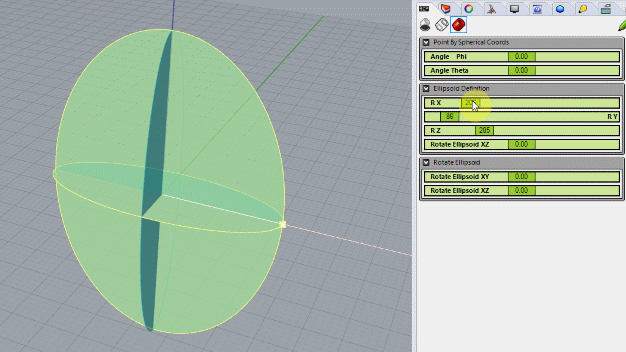# Tricky placing points on Ellipsoid with correct angles

OK, so I’ve been struggling to get correct position/angle for points on the surface of a tri-axial ellipsoid (three radii along X, Y and Z axes).

What I can do is to place points on the surface by entering angles `phi` (XY plane) and `theta` (Z-elevation).

What I cannot do is to get the points end up having correct position, that is, angles (as measured in euclidian World).

See examples of gross deviations pictured below. I do understand that Sin and Cos values tends to “drift” for angles between different radiuses, so I expect there are solutions out there with some additional formulas to compensate for this, but either I didn’t find any or I just didn’t recognize the solutions due to limited skills in math.

Fig 1. White (correct) angles manually drawn as 45º (135º) and 60º world XZ (rotation 180º and 0º respectively), but the spherical/ellipsoid angle/positions (red lines) ends up far off (“45” and “60”).

Fig 2. I also tried using Sphere.PointAt(Theta, Phi) with angle 45º (converted to radians) but also that results in gross deviation (58.179º as shown below).

I’ve spent several hours searching the Internet for a formula that would give correct positions, and although some say that “only approximations exist” for Ellipsoids (as opposed to Spheroids with two radiuses) I definitely expect to come closer than this.

I’d be fine with a radius length tolerance of, say ~0.05, and the same for position. I prefer a numerical solution for speed (intersecting a Line from a Spherical coordinate on a surrounding Sphere would be slow and a last resort).

The formula I have used;

``````        var theta = (Math.PI * 0.5) - Theta_AsRadians;
var cos_theta = Math.Cos(theta);
var sin_theta = Math.Sin(theta);

// XYZ  coordinate where RX, RY and RZ are the three axes, formula
// based on https://en.wikipedia.org/wiki/Ellipsoid#Parameterization
var x = RX * cos_theta * cos_phi;
var y = RY * cos_theta * sin_phi;
var z = RZ * sin_theta;

return new Point3d(x, y, z);
``````

As indicated, I’m not the math-guru guy you would rush to when in trouble, but if you have read the post this far, then perhaps you are the math-guru?TIA,

// Rolf

Hi Rolf

Well … obviously no math guru here …But what about a couple of curve-line intersections ?

http://developer.rhino3d.com/api/RhinoCommonWin/html/M_Rhino_Geometry_Intersect_Intersection_CurveLine.htm

First intersection on the XY plane ellipse using phi
The you build the ‘vertical isocurve’ ellipse and repeat the intersection using theta

ellipsoid.3dm (30.0 KB)

Maybe too slow ?

(Assuming the ‘algorithm’ is not completely wrong …)

Regards

Nice try, as usual.

The strategy works well. I tested by constructing a GH definition (GH attached below).

How fast it is I don’t really know, but I will try to make a “code version” of the definition and do some tests (the GH definition isn’t very fast though).

emilio’s_ellipsoid_reconstructed.gh (47.2 KB)// Rolf

Thanks for sharing the definition !

Very professional